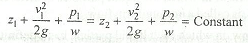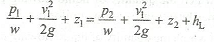Bernoulli's Equation

The Bernoulli's equation states that 'For a perfect incompressible liquid, flowing in a continuous stream, the total energy of a particle remains the same, while the particle moves from one point to another. Mathematically,The Bernoulli's equation is applied to venturimeter, orifice meter and pitot tube. The Bernoulli's equation for real fluids is given bywhere

hL = Loss of energy between sections 1 and 2.